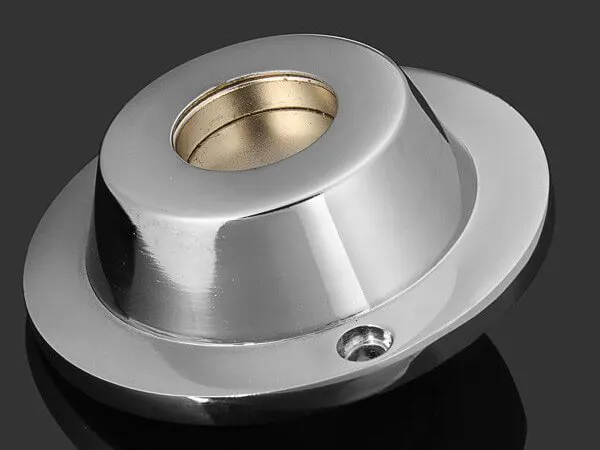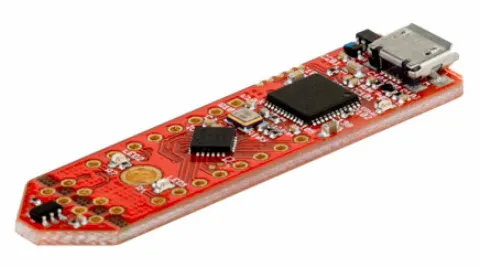# The Magnet Tag!

A funky cool looking high power magnet tag with a wide range of applications!

IntermediateProtip1 hour1,171## Things used in this project

### Hardware componentsInfineon 3D Magnetic Sensor 2Go
×1

### Software apps and online servicesArduino IDE

## Code

### Sample Code for Infineon Sensor

C/C++
It has most of the application codes I stated like the people counter, monitoring etc
```// Define includes
#include <Wire.h>        // Wire header file for I2C and 2 wire

//
int HallAddressWrite = 0x1F;   // Device address
byte X_Axis_Register1 = 0x1;  //

// Declare some variables
int const numOfBytes = 7;
int baudRate = 9600;
float counter=0.0;

byte configReg = 0x00;  // Address of Configuration
byte powerMode = 0x05;   // Set to low power mode

void setup() {
Serial.begin(baudRate);            // Set Serial Port speed
delay(1000);
Wire.pins(4, 5);
Wire.begin();                      // Join the I2C bus as a master

Wire.write(configReg);              // Address the Configuration register
Wire.write(powerMode);              // Set the Power Mode to Low
Wire.endTransmission();             // Stop transmitting
delay(100);
}

// Main Program Infinite loop
void loop() {

float temp = getTemp();
while(temp<-50){
temp = getTemp();
}
}
Serial.print("\t");
getMagX();
Serial.print("\t");
getMagY();
Serial.print("\t");
getMagZ();

Serial.println("-----");
delay(250);

}

float getFrameCounter() { //every ADC conversion the frame is incremented,this function captures that
//This would be needed probaly in fast mode to ensure data
//was succesfully written to the registries
int i;
int a;
int lsb = readByte;
int tableI = { 0, 0, 0, 0, 1, 1, 0, 0};

for ( i = 7; i >= 0; i--)
{
if ( (1 << i) & lsb)
a[7 - i] = 1*tableI[7-i];
else
a[7 - i] = 0;
counter += a[7 - i];
}
Serial.print(counter);
return counter;
}

float getTemp()
{
int i;
int a;
int b;
int tableI = { -2048, 1024, 512, 256, 0, 0, 0, 0};
int tableII = {128, 64, 32, 16, 8, 4, 2, 1};
float Celsius = 0;
int msb = readByte;
int lsb = readByte;

//msb conversion
for ( i = 7; i >= 0; i--)
{
if ( (1 << i) & msb)
a[7 - i] = 1 * tableI[7 - i];
else
a[7 - i] = 0;
//    Serial.print(a[7 - i]);Serial.print(" ");
Celsius += a[7 - i];
}
//  Serial.print("\t");
a = 0; // ascii terminating character

//lsb conversion
for ( i = 7; i >= 0; i--)
{
if ( (1 << i) & lsb)
b[7 - i] = 1 * tableII[7 - i];
else
b[7 - i] = 0;
//    Serial.print(b[7 - i]);Serial.print(" ");
Celsius += b[7 - i];
}
//  Serial.print("\t");
b = 0; // ascii terminating character

Celsius -= 320;
Celsius *= 1.1;
if(Celsius>-50){
Serial.print(Celsius);
}
return Celsius;

}

void getBytes(byte address, byte registry, int numOfBytes, byte* readByte)
{
//Ask for data register
Wire.write(registry);
Wire.endTransmission();             //End Transmission
delay(20);                        //at least 12msec for ADC conversion and storage
Wire.requestFrom(address, numOfBytes);     //Request Transmission
for (int i = 0; i < numOfBytes; i++) {
}
Wire.endTransmission();

}

float getMagX()
{
int i;
int a;
int b;
int tableI = { -2048, 1024, 512, 256, 128, 64, 32, 16};
int tableII = {8, 4, 2, 1, 0, 0, 0, 0};
float magX = 0;
int msb = readByte;
int lsb = readByte;

//msb conversion
for ( i = 7; i >= 0; i--)
{
if ( (1 << i) & msb)
a[7 - i] = 1 * tableI[7 - i];
else
a[7 - i] = 0;
//    Serial.print(a[7 - i]);Serial.print(" ");
magX += a[7 - i];
}
//  Serial.print("\t");
a = 0; // ascii terminating character

//lsb conversion
for ( i = 7; i >= 0; i--)
{
if ( (1 << i) & lsb)
b[7 - i] = 1 * tableII[7 - i];
else
b[7 - i] = 0;
//    Serial.print(b[7 - i]);Serial.print(" ");
magX += b[7 - i];
}
//  Serial.print("\t");
b = 0; // ascii terminating character

magX *= 0.098 * 10.0; //0.098mT/LSB 10Gauss/mT
if(abs(magX)<3)   //the sensor has about a 0.2mT | 2Gauss units drift
magX = 0;       //this is a software filter that suppress most of the noise
Serial.print(magX);
return magX;

}

float getMagY()
{
int i;
int a;
int b;
int tableI = { -2048, 1024, 512, 256, 128, 64, 32, 16};
int tableII = {0, 0, 0, 0, 8, 4, 2, 1};
float magY = 0;
int msb = readByte;
int lsb = readByte;

//msb conversion
for ( i = 7; i >= 0; i--)
{
if ( (1 << i) & msb)
a[7 - i] = 1 * tableI[7 - i];
else
a[7 - i] = 0;
//    Serial.print(a[7 - i]);Serial.print(" ");
magY += a[7 - i];
}
//  Serial.print("\t");
a = 0; // ascii terminating character

//lsb conversion
for ( i = 7; i >= 0; i--)
{
if ( (1 << i) & lsb)
b[7 - i] = 1 * tableII[7 - i];
else
b[7 - i] = 0;
//    Serial.print(b[7 - i]);Serial.print(" ");
magY += b[7 - i];
}
//  Serial.print("\t");
b = 0; // ascii terminating character

magY *= 0.098 * 10.0; //0.098mT/LSB 10Gauss/mT
if(abs(magY)<3)    //the sensor has about a 0.2mT | 2Gauss units drift
magY = 0;        //this is a software filter that suppress most of the noise
Serial.print(magY);
return magY;

}

float getMagZ()
{
int i;
int a;
int b;
int tableI = { -2048, 1024, 512, 256, 128, 64, 32, 16};
int tableII = {0, 0, 0, 0, 8, 4, 2, 1};
float magZ = 0;
int msb = readByte;
int lsb = readByte;

//msb conversion
for ( i = 7; i >= 0; i--)
{
if ( (1 << i) & msb)
a[7 - i] = 1 * tableI[7 - i];
else
a[7 - i] = 0;
//    Serial.print(a[7 - i]);Serial.print(" ");
magZ += a[7 - i];
}
//  Serial.print("\t");
a = 0; // ascii terminating character

//lsb conversion
for ( i = 7; i >= 0; i--)
{
if ( (1 << i) & lsb)
b[7 - i] = 1 * tableII[7 - i];
else
b[7 - i] = 0;
//    Serial.print(b[7 - i]);Serial.print(" ");
magZ += b[7 - i];
}
//  Serial.print("\t");
b = 0; // ascii terminating character

magZ *= 0.098 * 10.0; //0.098mT/LSB 10Gauss/mT
if(abs(magZ)<3) //the sensor has about a 0.2mT | 2Gauss units drift
magZ = 0;     //this is a software filter that suppress most of the noise
Serial.print(magZ);
return magZ;

}
```

## Credits

### Dhairya Parikh

21 projects • 113 followers
Project Developer | IoT and Machine Learning Enthusiast | Open Source Enthusiast |